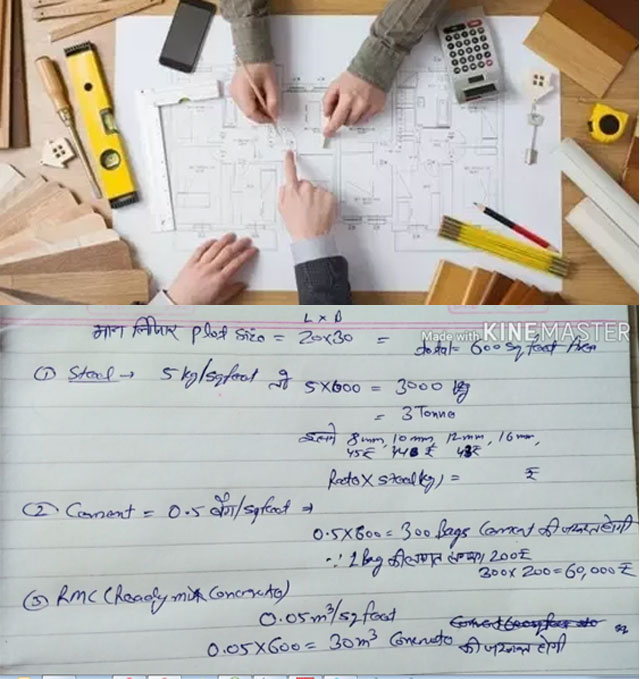# Different types of thumb rules for determining the total construction cost of the buildingThis construction video tutorial is very useful for site engineer. In this video, one can learn how to determine the construction cost of the entire building as well as the required quantities of materials on the basis of thumb rules.

### Given below the quantity and rates of materials per square feet :-

* Steel = 3 to 5 kg
* Cement = 0.5 bags
* RMC or ready mix concrete = 0.05 m3
* Block = 12.5 Nos/m2
* Electrical cost = Rs. 133
* Plumbing cost = Rs. 126
* Fire fighting cost = Rs. 40
* External development = Rs. 94.5
* Civil work structure = Rs. 751.25
* Finishing work = Rs. 467.50

Suppose, the length of the plot is given as 20 feet

The breadth of the plot is given as 30 feet

So, the size of the plot = Length x Breadth = 20 x 30 = 600 square feet

The requirement of steel (5 kg/sq.ft) = 5 x 600 = 3000 kg = 3 Tonne

It includes 8 mm, 10 mm, 12 mm and 16 mm dia steel. The rate of 8 mm is Rs. 45/kg, 10 mm is Rs. 44/kg, 12 mm is Rs. 48/kg etc.

So, the quantity of steel = rate x steel kg

The requirement of cement (0.5 bag/sq.ft) = 0.5 x 600 = 300 bags. The cost of 1 bag is Rs. 200 (approx).

The total cost of cement = 300 x 200 = Rs. 600

The requirement of RMC alias ready mix concrete (0.05 m3/sq.ft) = 0.05 x 600 = 30 m3 cement

The cost of electricity (Rs. 133/sq.ft) = 133 x 600 = Rs. 79,800

The cost of plumbing (Rs. 126/sq.ft) = 126 x 600 = Rs. 75,600

The cost of fire alarm (Rs. 40/sq.ft) = 40 x 600 = Rs. 24,000

The construction cost with labor (Rs. 751/sq.ft) = 751 x 600 = Rs. 4,50,600

The cost of finishing work (Rs. 467/sq.ft) = 467 x 600 = Rs. 2,80,200

In finishing work interior part, exterior part and paintings are included.

To gather knowledge on other types of thumb rules, go through the following video tutorial.